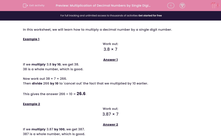# Multiplication of Decimal Numbers by Single Digit Numbers

In this worksheet, students must multiply one-digit numbers with up to two decimal places by whole numbers.Key stage:  KS 2

Curriculum topic:   Maths and Numerical Reasoning

Curriculum subtopic:   Decimals

Difficulty level:#### Worksheet Overview

In this worksheet, we will learn how to multiply a decimal number by a single digit number.

Example 1

Work out:

3.8 × 7

If we multiply 3.8 by 10, we get 38.

38 is a whole number, which is good.

Now work out 38 × 7 = 266.

Then divide 266 by 10 to 'cancel out' the fact that we multiplied by 10 earlier.

This gives the answer 266 ÷ 10 = 26.6.

Example 2

Work out:

3.87 × 7

If we multiply 3.87 by 100, we get 387.

387 is a whole number, which is good.

Now work out 387 × 7 = 2709.

Then divide 2709 by 100 to 'cancel out' the fact that we multiplied by 100 earlier.

This gives the answer 2709 ÷ 100 = 27.09.

### What is EdPlace?

We're your National Curriculum aligned online education content provider helping each child succeed in English, maths and science from year 1 to GCSE. With an EdPlace account you’ll be able to track and measure progress, helping each child achieve their best. We build confidence and attainment by personalising each child’s learning at a level that suits them.

Get started